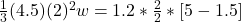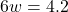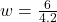## A uniform, 4.5 kg, square, solid wooden gate 2.0 mm on each side hangs vertically from a frictionless pivot at the center of its upper edge.

Question

A uniform, 4.5 kg, square, solid wooden gate 2.0 mm on each side hangs vertically from a frictionless pivot at the center of its upper edge. A 1.2 kg raven flying horizontally at 5.0 m/s flies into this door at its center and bounces back at 1.5 m/s in the opposite direction. What is the angular speed of the gate just after it is struck by the unfortunate raven?

in progress 0
6 months 2021-07-30T12:14:31+00:00 1 Answers 3 views 0

The angular velocity is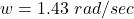Explanation:

From the question we are told that

The  mass of wooden gate  is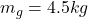The  length of side is  L = 2 m

The mass of the raven is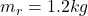The initial speed of the raven is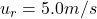The final speed of the raven is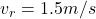From the law of  conservation of angular momentum we express this question mathematically as

Total initial angular momentum  of both the Raven and  the Gate =  The Final angular momentum of both the Raven and the Gate

The initial angular momentum of the Raven is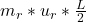Note: the length is half because the Raven hit the gate at the mid point

The initial angular momentum of the Gate is  zero

Note: This above is the generally formula for angular momentum of  square objects

The final angular velocity  of the Raven is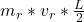The  final angular velocity of the Gate  is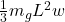Substituting this formula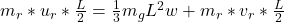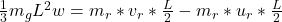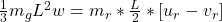Where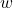is the angular velocity

Substituting value